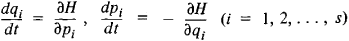# Hamilton's Canonical Equations of Motion

The following article is from The Great Soviet Encyclopedia (1979). It might be outdated or ideologically biased.

## Hamilton’s Canonical Equations of Motion

the differential equations of motion of a mechanical system in which the variables are the generalized momenta pi, as well as the generalized coordinates qi; the qi and pi, are called canonical variables. Hamilton’s canonical equations of motion have the formwhere H (qi, pi, t) is the Hamiltonian function, which when the constraints are not time-dependent and the acting forces are potential is equal to the sum of the kinetic and potential energies of the system expressed in terms of canonical variables, and s is the number of degrees of freedom of the system. By integrating this system of ordinary first-order differential equations it is possible to find all the qi and pi as functions of the time t and 2s constants, which are determined from the initial conditions.

Hamilton’s equations have the important property that they make it possible, using canonical transformations, to transform from the qi and pi to new canonical variables Qi (qi, pi, t) and Pi (qi, pi, t ) that also satisfy Hamilton’s equations, but with a different function H (Qi, Pi, t). In this manner, Hamilton’s equations can be reduced to a form that simplifies the process of integration. Hamilton’s equations are used in statistical physics, quantum mechanics, electrodynamics, and other branches of physics, as well as in classical mechanics.

S. M. TARG